5.1 - Angles of Triangles
5.2 - Congruent Polygons
5.4 - Equilateral and Isosceles Triangles
5.3/5.5/5.6 Proving Triangles Congruence
Some Potpourri
100

Solve for xx = 109

100

Name a pair of congruent sides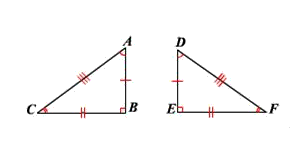AB and DE

BC and EF

CA and FD

100

Solve for x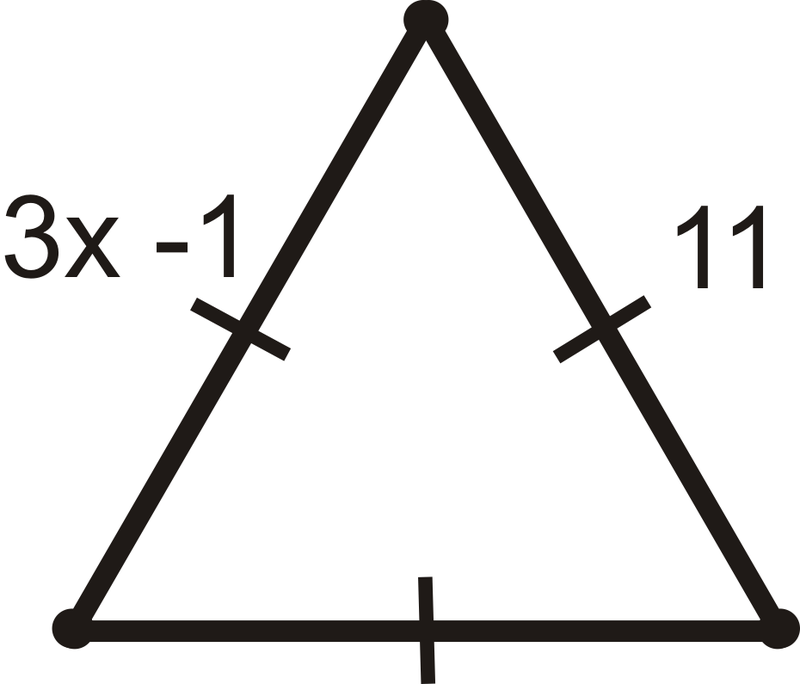x = 4

100

Are these two triangles congruent? If so, by which theorem?Yes, by SSS

100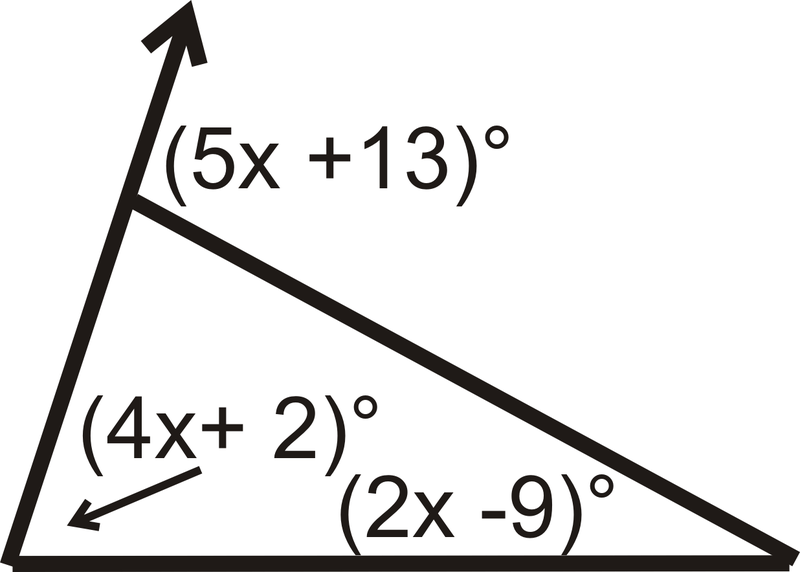x = 20

200

Solve for the missing angle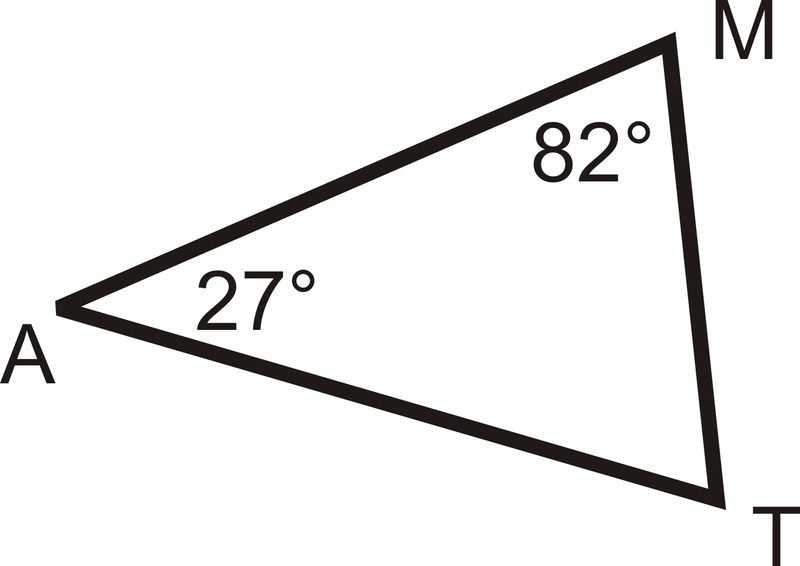T = 71 degrees

200

Name a pair of congruent anglesAngle A and Angle D

Angle B and Angle E

Angle C and Angle F

200

Solve for a if DE = 5a - 3, EF = 2a + 6, and DF = 7a - 12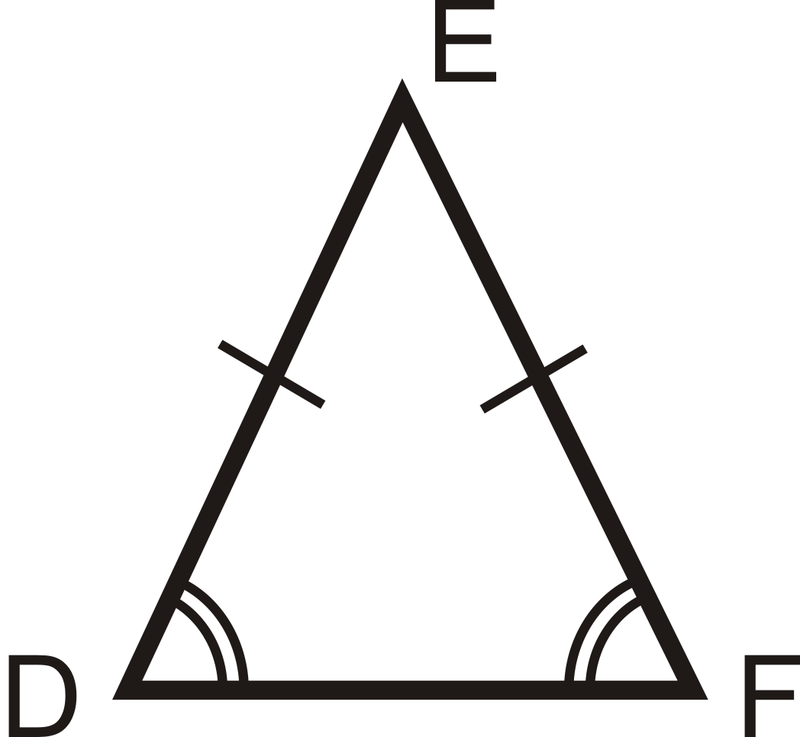a = 3

200

Are these triangles congruent? If so, by which theorem?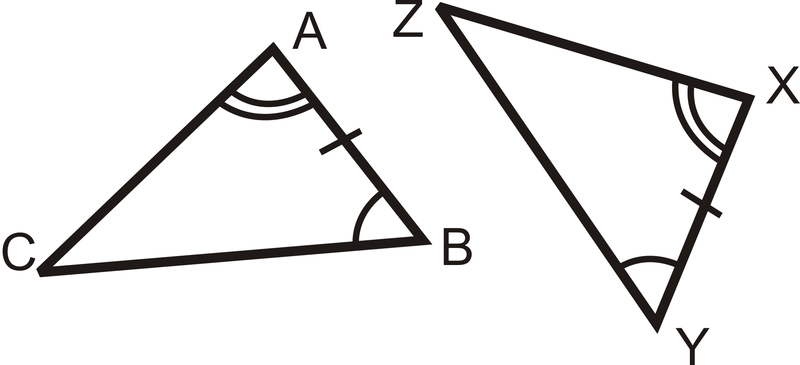Yes, by ASA

200

Solve for x: Angle 1 = 7x + 12, Angle 2 = 3x + 14, Angle 3 = 3x - 15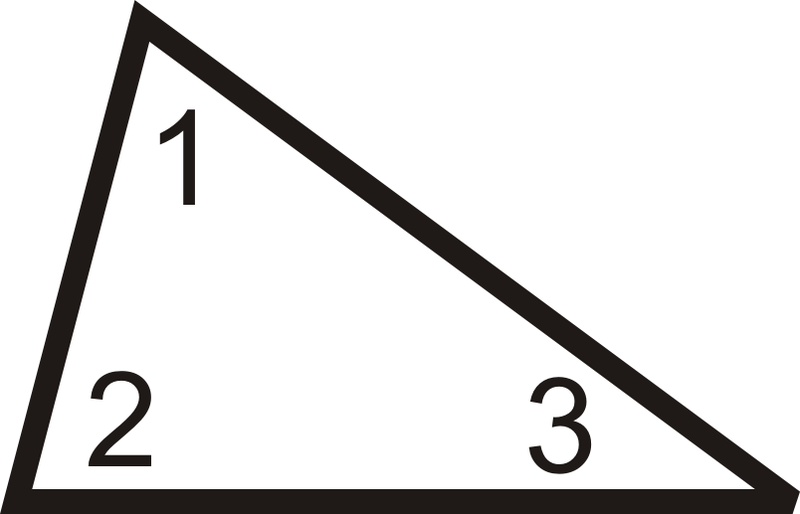x = 13

300

Solve for yy = 10

300

If ABCD is congruent to EFGH and AB = 3x + 4 and EF = 25, solve for x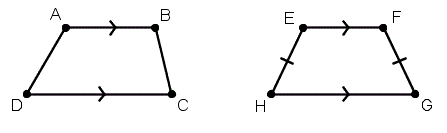x = 7
300

Solve for x and y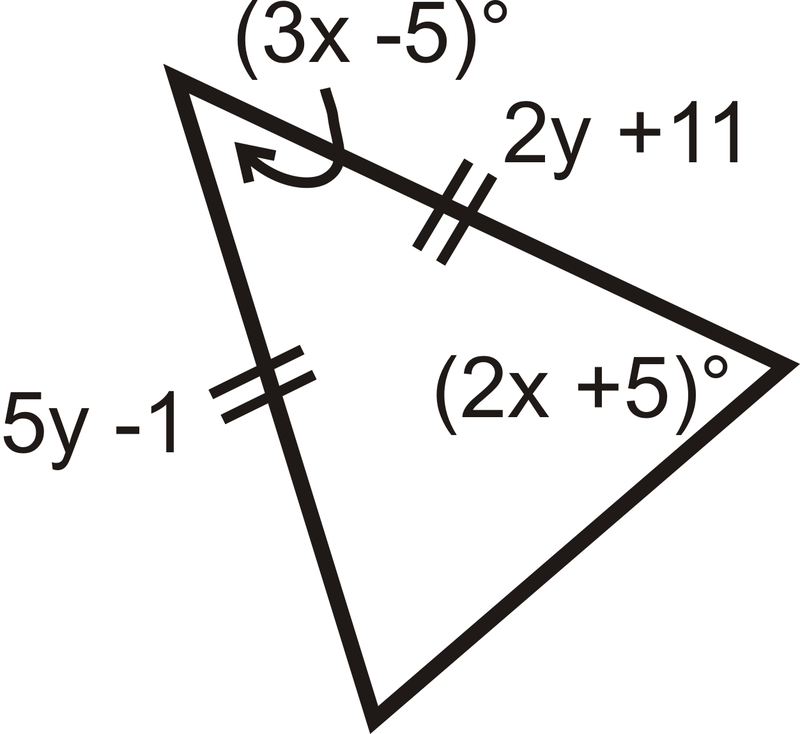x = 25

y = 4

300

Can you prove these two triangles are congruent? By which theorem?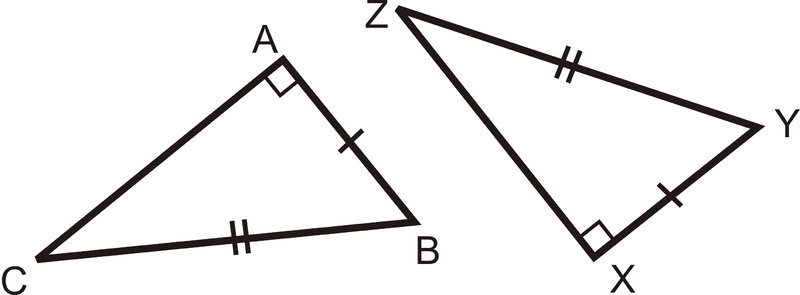Yes, by HL

300

Segment AB = 13x - 4, Segment BC = 9x + 24, Segment AC = 6x + 2x = 7

400

Solve for x AND classify the triangle by its angles.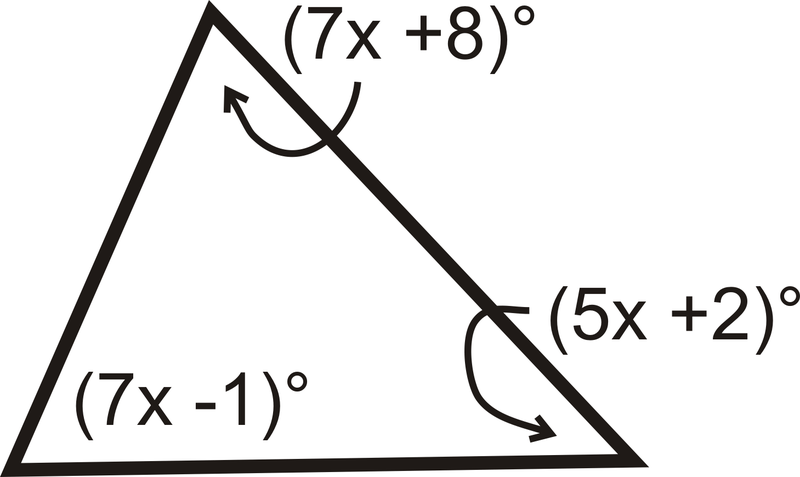x = 9

Acute Triangle

400

Solve for x if we assume BAHG is congruent to DCFE

NOTE: 4x + 5 is on Angle Hx = 25

400

Solve for x

NOTE: Line GF should say 3x + 10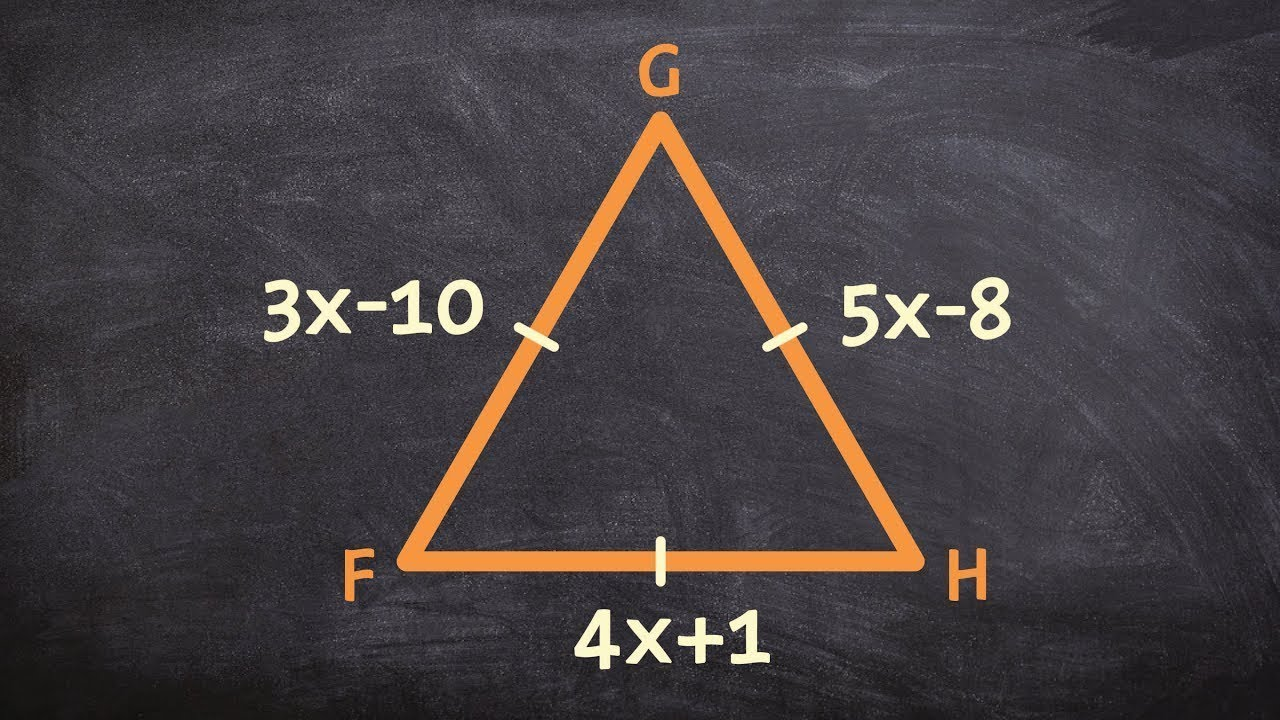x = 9

400

Write a congruent statement and state the theorem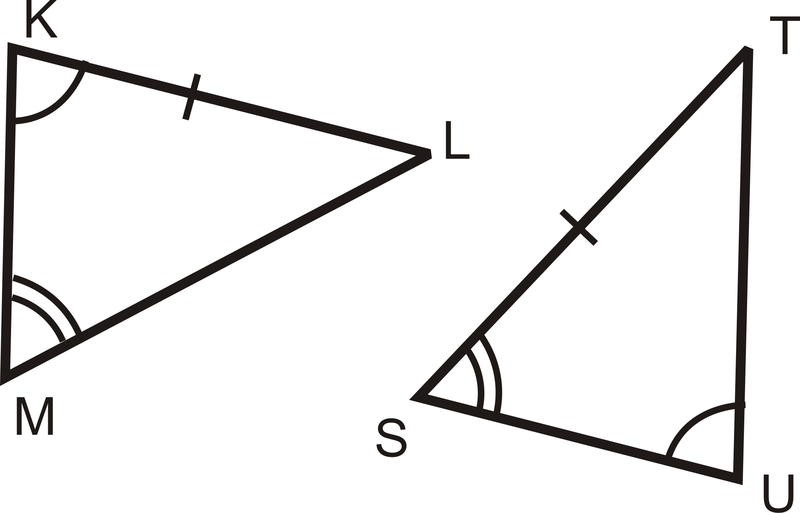2) By AAS

400

Are they congruent? Prove itYes, by SSS

500

Solve for x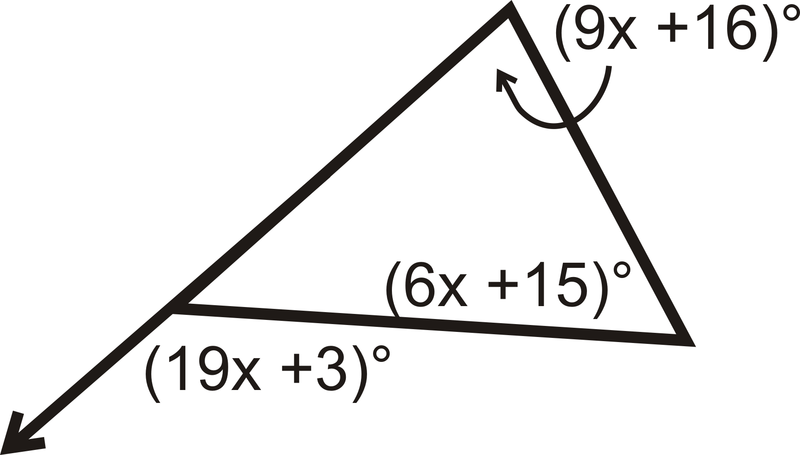x = 7
500

Write a congruent statement of the image below500

Assume: Triangle ABC is congruent to Triangle DFE

Solve for x and y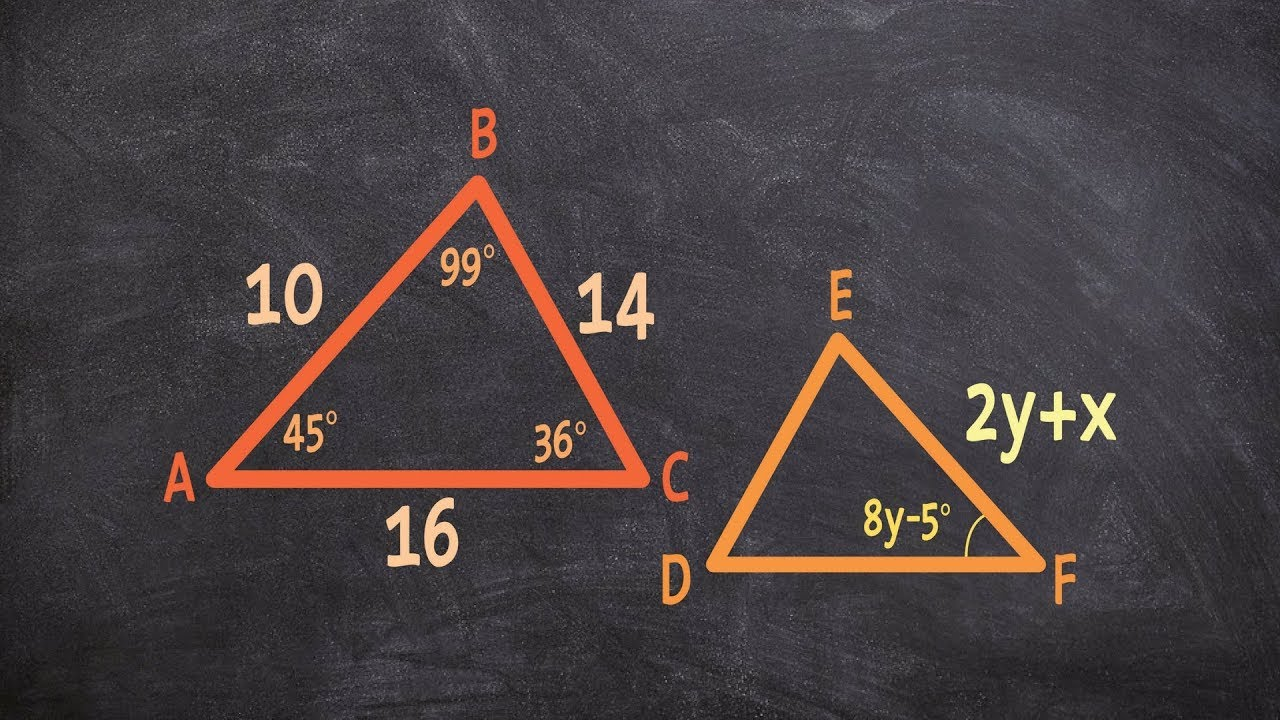x = -12

y = 13

500

Give a congruent statement to the two triangles and identify the theorem that makes the two congruent.2) by ASA

500

Fill in the blank

Corresponding

P ____________ of

Congruent

T ____________ are

C ____________

Parts

Triangles

Congruent

Click to zoom